# Boyle's Law

Back to Gas Laws

### Boyle's Law

Boyle's Law states that the volume of a gas is inversely proportional (inversely related) to it's pressure. In other words, as the pressure goes up, the volume goes down. Pressure squeezes the gas molecules closer together, so the volume becomes smaller. See the animation on the right for a visual idea of how increasing the pressure (the green weights on the lid) affects the volume.

Boyle’s law states that the product of the pressure and volume of a gas is a constant (Equation #1, below).  The value of that constant is unimportant to us, and depends upon the units used to measure the pressure and volume.  However, it is very useful to know that the product is a constant, because that means if pressure goes up, volume must go down.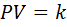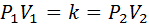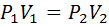Equation #1 Equation #2 Equation #3

We can use Equation #1 and some mathematical magic (the transitive property, Equation #2) to make Equation #3.  Basically, if the product of the pressure and volume at one condition (P1V1) equals a constant, then it also equals that same constant at some other condition (P2V2).

We can rearrange Equation #3 to solve for any of the four variables.  For instance, if we want to solve for the final pressure (P2) after changing the volume from V1 to V2, the equation becomes,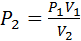Equation #4

This equation works with any units for pressure and volume, as long as the units in the first and second conditions are the same.  If the units in 1 & 2 are different, one set will need to be converted.

Consider the example word problem, below:

A piston in the engine of a sports car has a volume of 1.50 liters.  During compression its volume decreases to 0.25 liters.  If the initial pressure is 735 mmHg, what is the final pressure in atmospheres?

First, identify the variables.  I typically require my students to list them down the left side of their worksheets. In this problem, P1 =735 mmHg, V1 = 1.50 L, V2=0.25 L, and P2 is the unknown.  The problem asks for the answer in atmospheres, so we must decide if we want to convert 735 mmHg to atmospheres first, or convert the answer to atmospheres later.  It really doesn’t matter.  In this example, we will convert later.

We already solved the equation above (#4) for P2, so all we need to do is substitute values.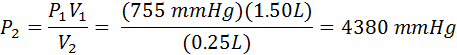Now we need to convert our final answer to atmospheres.  We need to look at our pressure conversions for that.  We find that 1.00 atm = 760 mmHg, so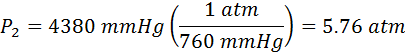Again, Equation #3 can be solved for any of the 4 variables as it was for P2 in Equation #4.  If necessary, students can substitute directly into Equation #3 first, and then solve for the unknown variable.

#### Practice

Click on the image above to go go to a site where you can practice Boyle's Law probems in an infinite number of combinations